# Search by Topic

#### Resources tagged with Complex numbers similar to Diagonals for Area:

Filter by: Content type:
Age range:
Challenge level:

### There are 21 results

Broad Topics > Numbers and the Number System > Complex numbers### Three by One

##### Age 16 to 18 Challenge Level:

There are many different methods to solve this geometrical problem - how many can you find?### 8 Methods for Three by One

##### Age 14 to 18 Challenge Level:

This problem in geometry has been solved in no less than EIGHT ways by a pair of students. How would you solve it? How many of their solutions can you follow? How are they the same or different?. . . .### What Are Complex Numbers?

##### Age 16 to 18

This article introduces complex numbers, brings together into one bigger 'picture' some closely related elementary ideas like vectors and the exponential and trigonometric functions and. . . .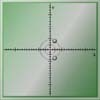### Conjugate Tracker

##### Age 16 to 18 Challenge Level:

Make a conjecture about the curved track taken by the complex roots of a quadratic equation and use complex conjugates to prove your conjecture.### Two and Four Dimensional Numbers

##### Age 16 to 18 Challenge Level:

Investigate matrix models for complex numbers and quaternions.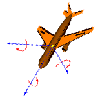### Complex Rotations

##### Age 16 to 18 Challenge Level:

Choose some complex numbers and mark them by points on a graph. Multiply your numbers by i once, twice, three times, four times, ..., n times? What happens?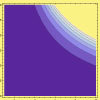### Sextet

##### Age 16 to 18 Challenge Level:

Investigate x to the power n plus 1 over x to the power n when x plus 1 over x equals 1.### Target Six

##### Age 16 to 18 Challenge Level:

Show that x = 1 is a solution of the equation x^(3/2) - 8x^(-3/2) = 7 and find all other solutions.### Sheep in Wolf's Clothing

##### Age 16 to 18 Challenge Level:

Can you work out what simple structures have been dressed up in these advanced mathematical representations?### Pumping the Power

##### Age 16 to 18 Challenge Level:

What is an AC voltage? How much power does an AC power source supply?### Complex Partial Fractions

##### Age 16 to 18 Challenge Level:

To break down an algebraic fraction into partial fractions in which all the denominators are linear and all the numerators are constants you sometimes need complex numbers.### Complex Sine

##### Age 16 to 18 Challenge Level:

Solve the equation sin z = 2 for complex z. You only need the formula you are given for sin z in terms of the exponential function, and to solve a quadratic equation and use the logarithmic function.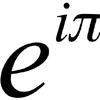### An Introduction to Complex Numbers

##### Age 16 to 18

A short introduction to complex numbers written primarily for students aged 14 to 19.### Thousand Words

##### Age 16 to 18 Challenge Level:

Here the diagram says it all. Can you find the diagram?### What Are Numbers?

##### Age 7 to 18

Ranging from kindergarten mathematics to the fringe of research this informal article paints the big picture of number in a non technical way suitable for primary teachers and older students.### Footprints

##### Age 16 to 18 Challenge Level:

Make a footprint pattern using only reflections.### Roots and Coefficients

##### Age 16 to 18 Challenge Level:

If xyz = 1 and x+y+z =1/x + 1/y + 1/z show that at least one of these numbers must be 1. Now for the complexity! When are the other numbers real and when are they complex?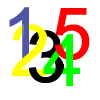### Complex Countdown

##### Age 16 to 18 Challenge Level:

Play a more cerebral countdown using complex numbers.### Napoleon's Theorem

##### Age 14 to 18 Challenge Level:

Triangle ABC has equilateral triangles drawn on its edges. Points P, Q and R are the centres of the equilateral triangles. What can you prove about the triangle PQR?### Impedance Can Be Complex!

##### Age 16 to 18 Challenge Level:

Put your complex numbers and calculus to the test with this impedance calculation.### Cube Roots

##### Age 16 to 18 Challenge Level:

Evaluate without a calculator: (5 sqrt2 + 7)^{1/3} - (5 sqrt2 - 7)^1/3}.